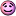Hi.
I have been given a function which reads off a pgm image into C++ which I'm having problem with. It seems that it can only read pgm files beginning with P2, and since I don't know good ways to convert any image to P2, i only used imread function on matlab and copy and paste the values into a text document, then saving it as a pgm file.
However, It still doesn't work. It works however, on the demo pgm image, which was made by paint shop pro.
I'm unsure if it is the problem of C++ code, or my image. If someone can help it would be greatly appreciated. I do have a suspicion that because it is copy and pasted from matlab, the spacing between each number affects the reading in C++, though i can open the image through inkscape. i.e
198 160 133 28 233 55 0 0 rather than 5 255 255 253 253
The codes are
#if !defined(IMAGE_H)
#define IMAGE_H

#include <vector>
#include <fstream>
#include <math.h>

struct image
{
image() : W(0), H(0) {}
image(int w, int h) : W(w), H(h) {buf.resize(W * H);}
~image() {}
bool empty() const {return buf.empty();}
const unsigned char& operator()(int x, int y) const {return buf[x + y * W];}
{
std::ifstream s(fn.c_str());
if(!s)
return false;
char a;
s >> a;
if (a != 'P')
return false;
s >> a;
if (a != '2')
return false;
char b;
s.getline(b, 4999);
s.getline(b, 4999);
int X;
s >> W >> H >> X;
if (X != 255)
return false;
buf.resize(W * H);
std::vector<unsigned char>::iterator p = buf.begin();
for (int i = 0; i < W * H; i++)
{
s >> X;
*p++ = (unsigned char)X;
}
return true;
}
void writePGM(std::string fn)
{
std:fstream s(fn.c_str());
if(s)
{
s << "P2\n#output from hocrtest\n" << W << " " << H << "\n" << (int)255 << "\n";
int c = 0;
while (c < W * H)
{
for (int i = 0; i < 19 && c < W * H; i++)
s << (int)buf[c++] << " ";
s << "\n";
}
}
}
void gaussianblur(image& blurred, double sigma) const
{
blurred.buf.resize(W * H);
int r = int(sigma * 3) + 1;
double s2 = sigma * sigma * 2;
for (int y = 0; y < H; y++)
for (int x = 0; x < W; x++)
{
double s = 0;
for (int v = -r; v <= r; v++)
{
int v1 = std::max(0, std::min(H - 1, y + v));
for (int u = -r; u <= r; u++)
{
int u1 = std::max(0, std::min(W - 1, x + u));
s += exp(-(u * u + v * v) / s2) * buf[v1 * W + u1];
}
}
blurred.buf[y * W + x] = (unsigned char)std::min(255.0, std::max(0.0, (s / s2 / 3.141592653589)));
}
}
int W, H;
std::vector<unsigned char> buf;
};

#endif